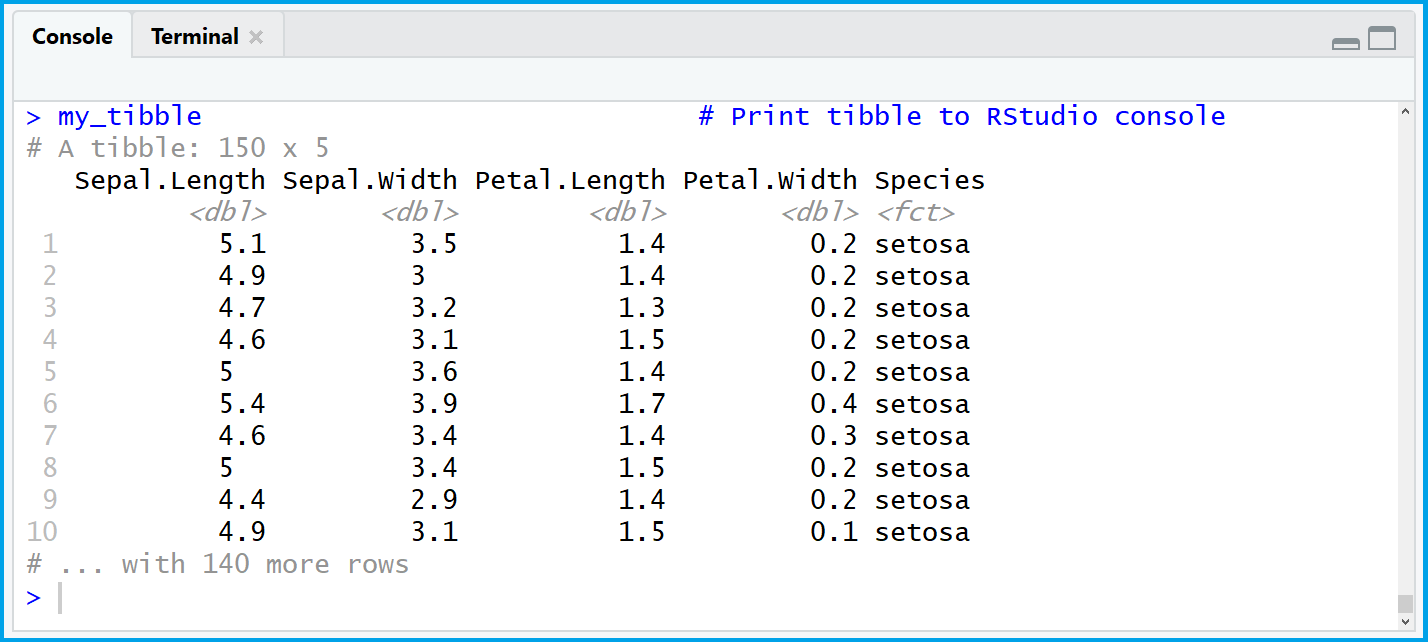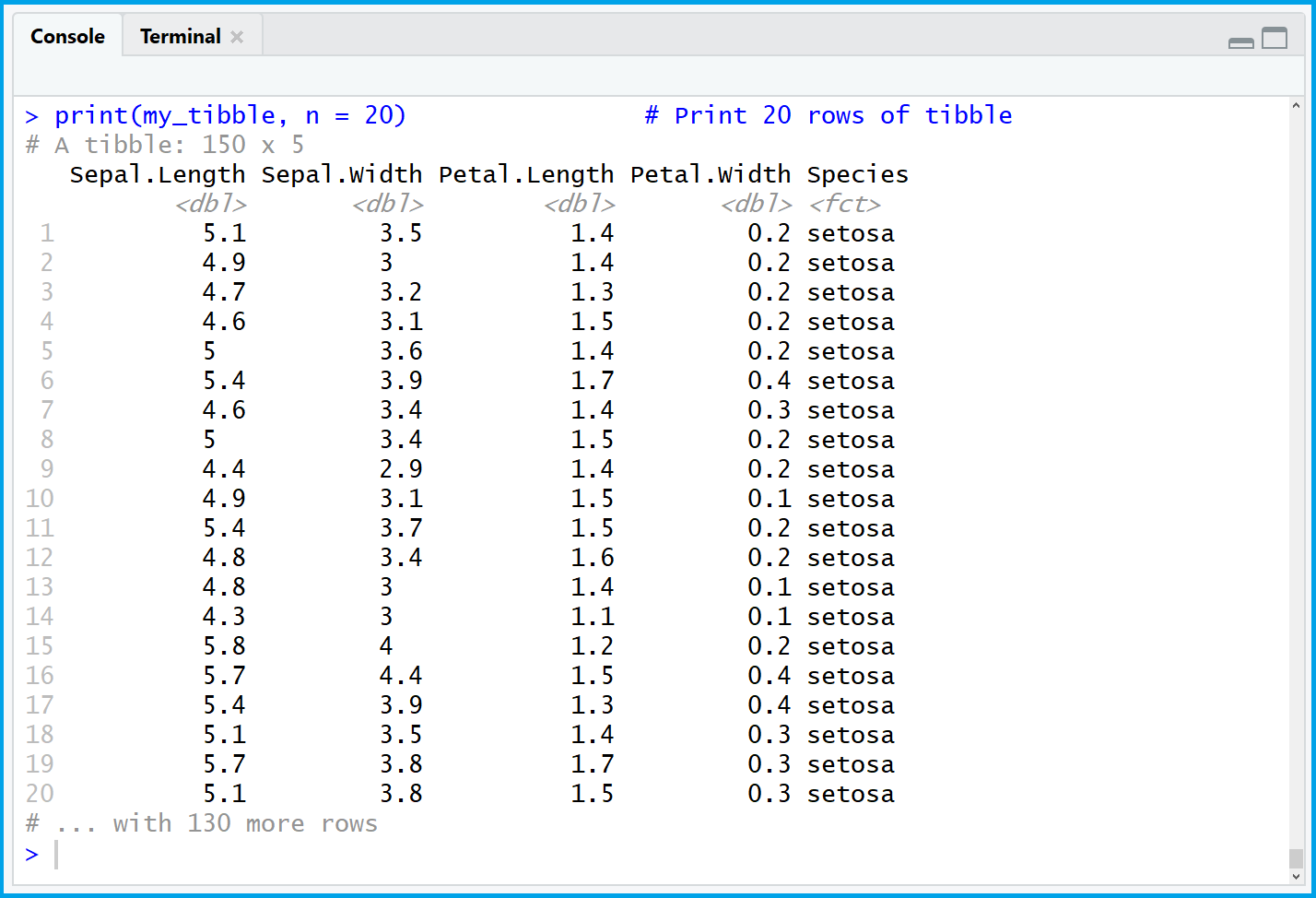Print Entire tibble to R Console (2 Examples)

In this tutorial you’ll learn how to print a tibble to the console in the R programming language.

The tutorial is structured as follows:

Here’s the step-by-step process.

Creation of Example Data

Most tibble (previously called tbl_df) operations are based on the dplyr package. Let’s install and load the dplyr package to R:

 install.packages("dplyr") # Install dplyr package library("dplyr") # Load dplyr package

Now, we can convert the iris data frame to a tibble with the as_tibble function of the dplyr package:

 my_tibble <- as_tibble(iris) # Create example tibble my_tibble # Print tibble to RStudio consoleTable 1: tibble Printed to Console with Default Specifications.

Table 1 illustrates how our example tibble is returned if we print it to the RStudio console – By default, only the first 10 rows of the data are shown.

In the following two examples, I’ll show you how to show a certain number or even all rows of your tibble in the RStudio console. So keep on reading!

Example 1: Display N Rows of tibble

If we want to display a specific amount of rows in the RStudio console, we can use the print() function as follows:

 print(my_tibble, n = 20) # Print 20 rows of tibbleTable 2: Printing 20 Rows of tibble.

Table 2 illustrates the RStudio console output of the previous R code. As you can see, we returned the first 20 rows of our tibble to the console by specifying n = 20.

Next; I’ll show you how to print an entire tibble to the console…

Example 2: Display All Rows of tibble

If we want to print our complete tibble to the console, we can simply use the print function in combination with the nrow function. Have a look at the following R syntax:

 print(my_tibble, n = nrow(my_tibble)) # Print all rows of tibble

If you execute this code in your RStudio, you will see that all of our tibble is returned in the console.

Video & Further Resources

I have recently published a video on my YouTube channel, which explains the topics of this article. You can find the video below.

In addition, you may have a look at the related tutorials on this website. I have released numerous posts about tibbles, the nrow command, or the RStudio console already: